## Introduction

In our daily life, there are many situations where we need to compare two quantities.

• When you want to know which is greater.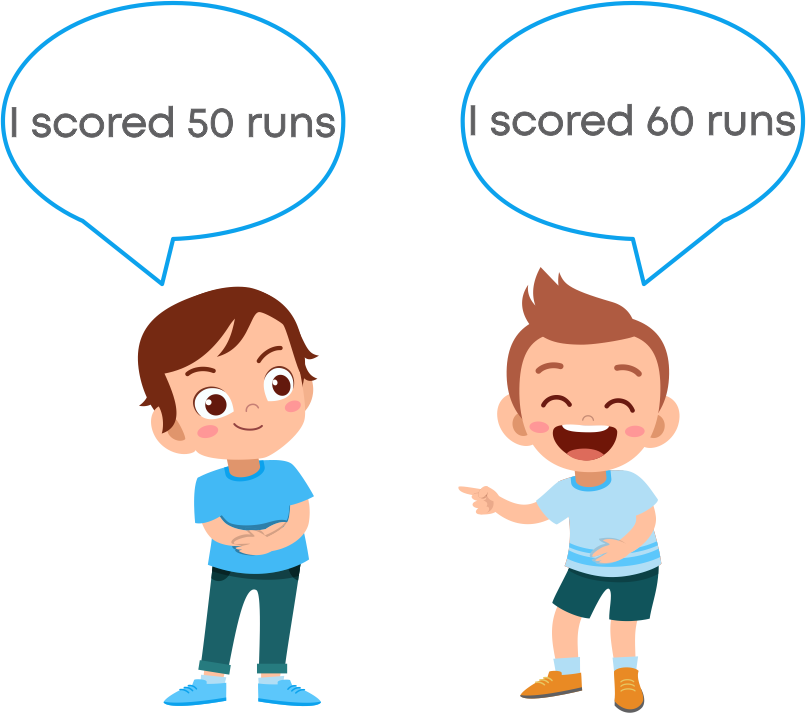• When you want to find an increase in quantity

For e.g., to calculate the increase in the population of the country, we compare the population of the current year to the previous year.

We use different ways to compare quantities. What are those different ways? Watch the video to find out.

It becomes easier to compare quantities when the quantities are in the form of a percentage.

Per cent means per 100. 32% means 32 per every 100. The percentage is the numerator of a fraction with denominator 100. We use the symbol ‘%’ to represent percentage.

Anu scored 80 marks out of 100 in the maths exam. How do we write Anu’s marks? Anu’s marks can be written as 80 100 . The percentage of Anu’s marks = 80%.

Percentages are used widely in our day-to-day life.

• Exam marks are converted in percentages for better understanding
• Profit and loss are always given as a percentage
• Interest in banks is always given as percentages
• Discount in shops during the sale is always given as percentages

#### Concepts

The chapter ‘Comparing Quantities’ covers the following concepts:

#### Ratios and Percentages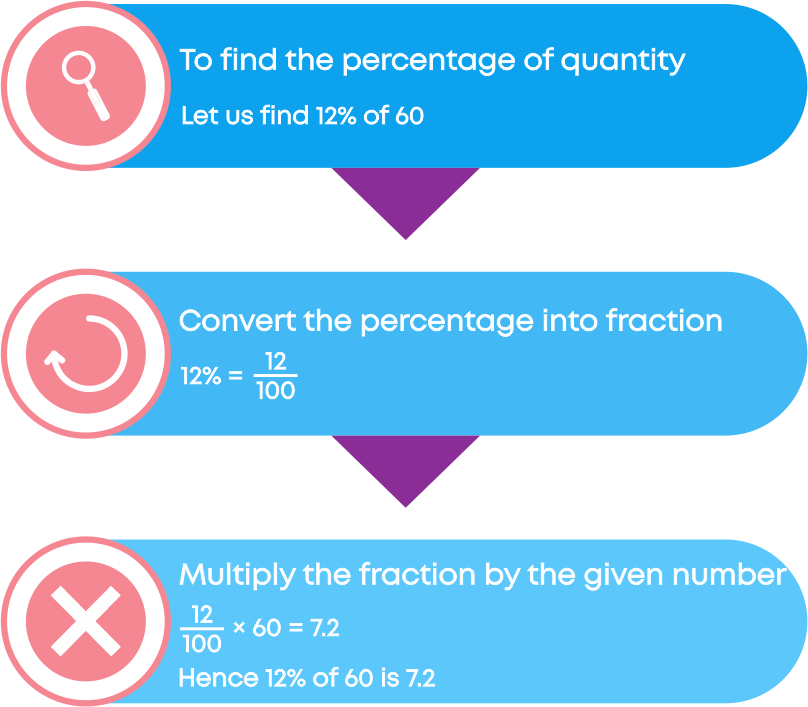We know how to find the percentage of a quantity. But how do we find the whole quantity if the percentage of the quantity is given? Let us take an example.

15% of 200 = 15 100 × 200 = 30

15% of 200 is 30 which means if 15% of the number is 30, then the number is 200. Now how do we find the number, if 15% of it is 30? Let the required number be ‘p’. We have 15% of ‘p’ as 30. We write 15% as 15 100

Hence, 15 100 × p = 30

p = 30 × 100 15 = 200

Hence, 15% of 200 is 30.

#### Discounts

Vivek went shopping with his mother. In a shop, he saw a bag that he wanted to buy. He also saw a price tag of ₹1000 on it. This is called the marked price of the product. At times it is also referred as listed price.Since it is sale season, the shopkeeper informed Vivek that he would get the bag for ₹700. The seller was selling the bag for ₹700. Here, ₹700 is the selling price of the bag. Vivek got excited as he could get the bag for ₹300 less than the actual price. Or we can say he would get a discount of ₹300. Discount is the reduction given on the marked price of the product.

Sellers often give discount:

• To attract customers to their stores.
• To dispose of their old stock.

The price at which a seller bought an item or product is called the cost price (CP).

The price on the label/tag of a product/item is called the marked price or list price.

The reduction in the marked price of an item offered by the seller is called discount.

The price at which the product is sold to a customer after the discount (if discount is given) is called selling price (SP).

Discount is the decrease in the marked price of an item.

So, the difference in the marked price and the selling price gives the discount offered on a product. Hence,The percentage of decrease in the marked price is known as discount %.

Two friends, Akshay and Manoj, are discussing the new bicycle they bought.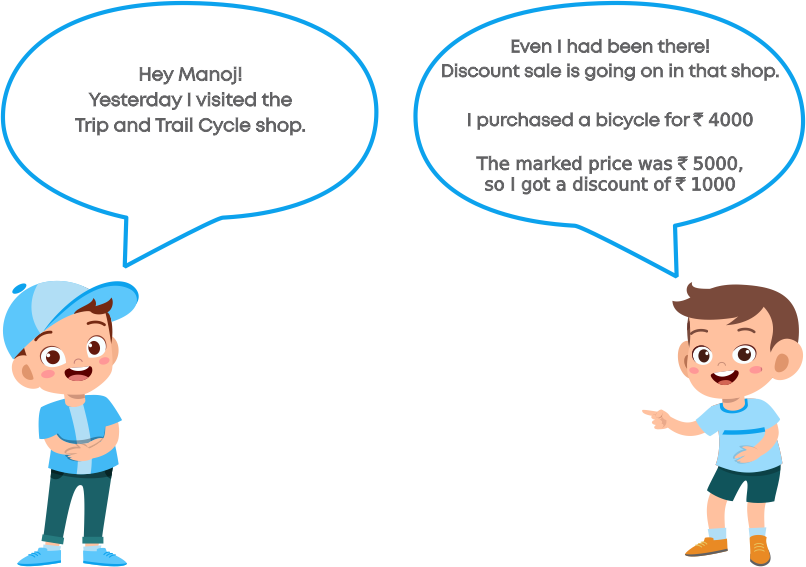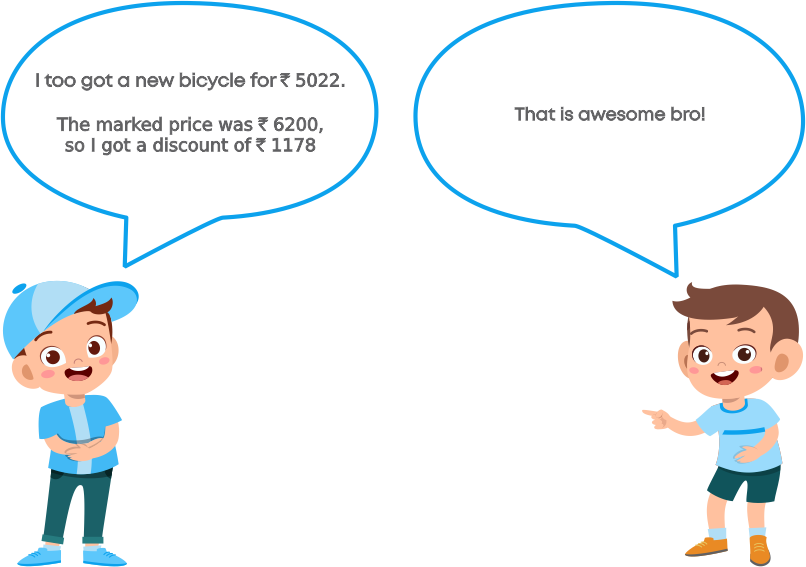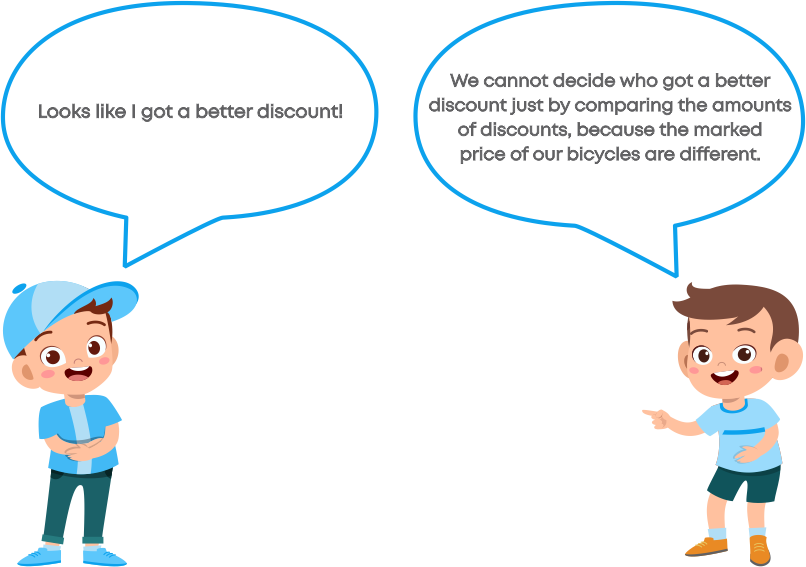Do you think Manoj is right? Yes. To compare who got a better discount, we need to find the discount per cent in each case.

We know that a discount is always calculated on the marked price of an item. If a discount of ₹200 is given on a shirt which is marked at ₹1000, how do we calculate the discount per cent?

To find the discount per cent, we need to find what per cent of ₹1000 is ₹200.

200 1000 × 100% = 200 10 % = 20%

Hence, the discount per cent is 20%.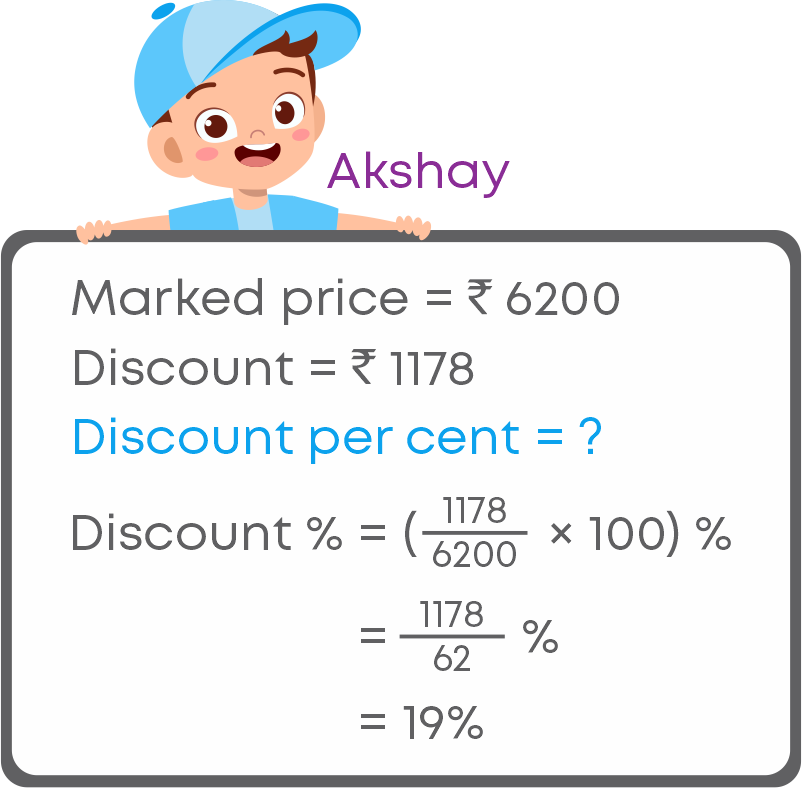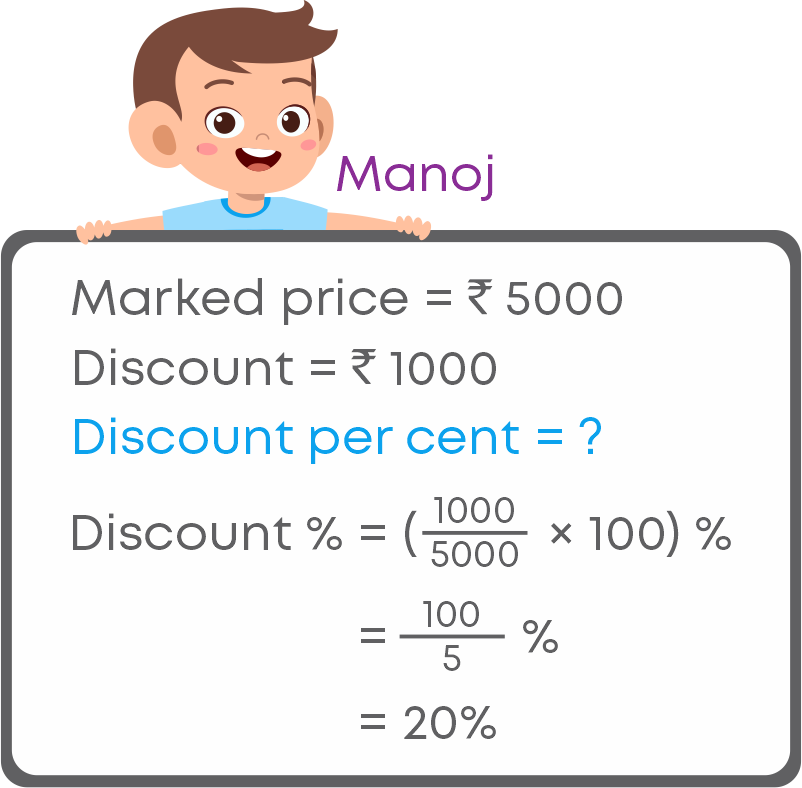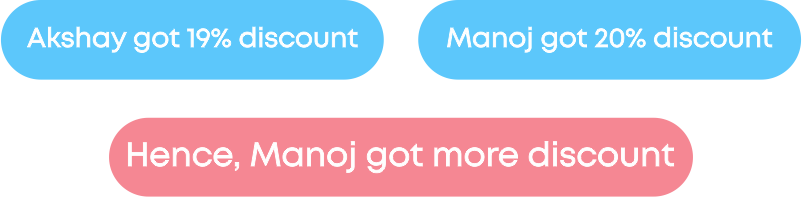So, discount % = ( discount marked price × 100)%

To solve problems on profit and loss, understand the question, identify what is given and what is to be found.

If you are asked to find profit % or loss %:

• Use the given formulas to devise the strategy to solve the problem:

profit % = profit CP × 100

loss % = loss CP × 100

If you are asked to find CP or SP, always find profit or loss on CP. Use the given formulas to devise the strategy to solve the problem:

• Loss = Cost price – Selling price
• Profit = Selling price – Cost price

To solve real-life problems based on discount follow the strategy below:

• Analyse the question to understand what is given and what we must find.
• Identify the keyword: Over and above
• 20% discount over and above the 10% discount means = 10% discount is given on the item, then on the reduced/discounted price 20% discount is given.
• Calculate the reduced price after the discount
• Then on the reduced price calculate the discount and find the final selling price.

Remember these keywords:

Off season means

• The time of the year that is less busy
• The season when there is less business

Over and above means

Successive means

• Following immediately
• One after the other

Consider this example: A game CD was marked for ₹4,650 and a discount of 18% was given for the year-end sale. Find the selling price.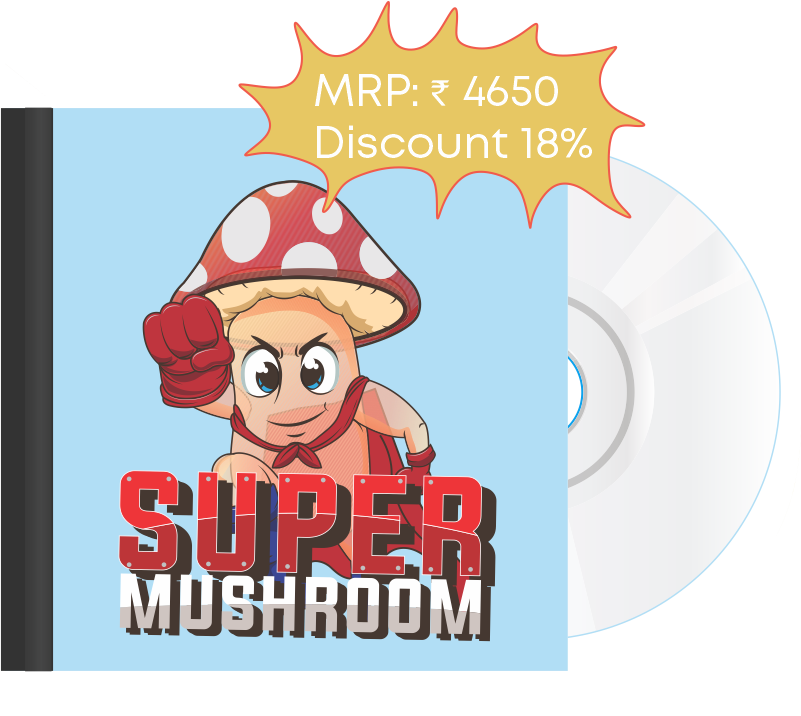We know that discount = marked price – selling price. Hence,

• Selling price = Marked price - Discount

To find the selling price we need to first find the discount. How do we find the discount given on the game CD? We know that the discount is always calculated as a per cent of the marked price. Hence, 18% of ₹4650

₹( 18 100 × 4650) = ₹(18 × 46.50) = ₹837

Hence, the discount is ₹837. Let us find the selling price using the formula

Discount = Marked price – Selling price

= ₹4650 - ₹837

= ₹3813

Hence, the selling price of the game CD is ₹3,813. So, to buy the game CD, we must pay ₹3,813.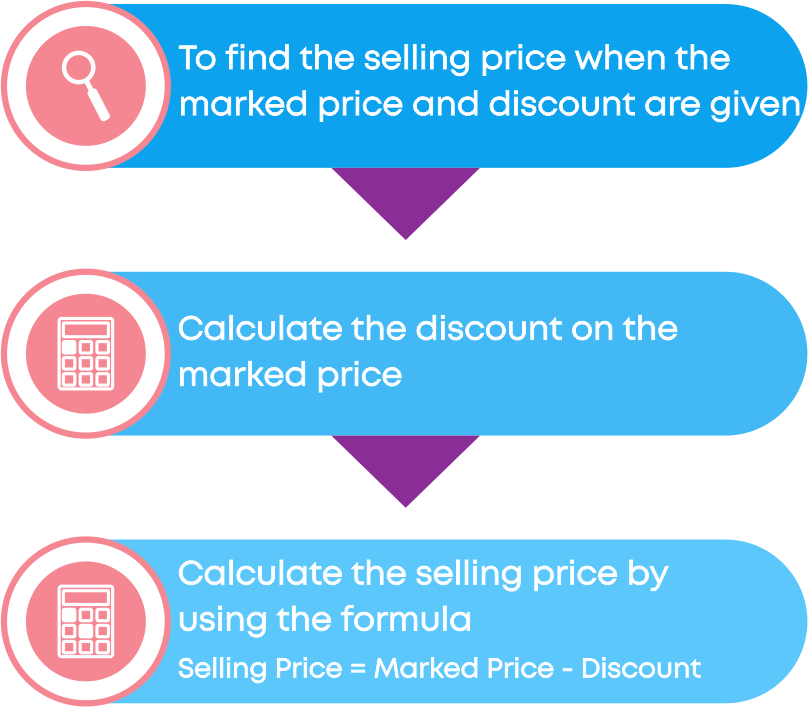To find the marked price of an item when the selling price and discount % are given follow these steps:

• Take the marked price as ₹100.
• Find the selling price when the marked price is ₹100.
• Perform cross multiplication to find the marked price for the given selling price.

#### Goods and Service Tax

Nisha bought a few items from a shop. The shopkeeper gave her the bill.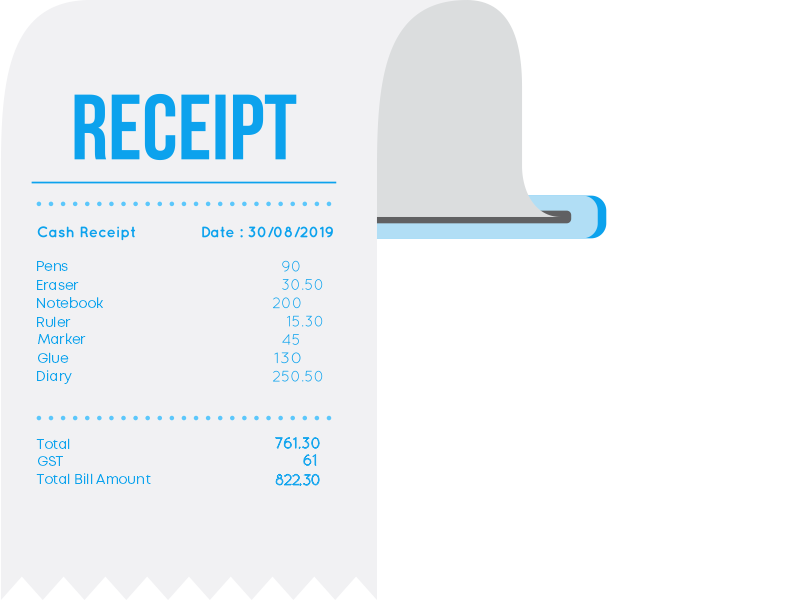She sees that she also must pay money which is added in the name of GST to the bill. Nisha is confused. So, she asks the shopkeeper. He tells her that it is the tax. Nisha has no clue about tax. Let us help Nisha understand what tax or GST is.

There are many kinds of taxes. Tax is money that people must pay to the government. The government uses the money to provide services such as education and health care and to maintain or build things like roads, bridges, and sewers. GST is a type of tax.

Goods and Services Tax (GST) is a tax that customers must pay when they buy any goods or services, such as food, clothes, items of daily needs, transportation etc.

For e.g., if Jyothi is buying a mixer, as a customer she needs to pay the base price of the mixer and GST to the shopkeeper. This GST will go to the government.

The price of a product is always written on the product as the MRP.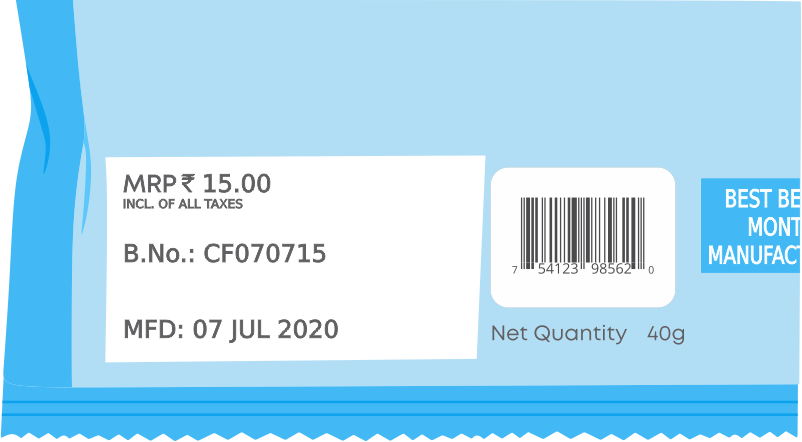MRP means the Maximum Retail Price. MRP is the maximum price for which the product can be sold. That is the product cannot be sold for more than the MRP. Hence, MRP is always inclusive of GST.

GST is always calculated as the fixed per cent of the base price. GST per cent is different for different categories.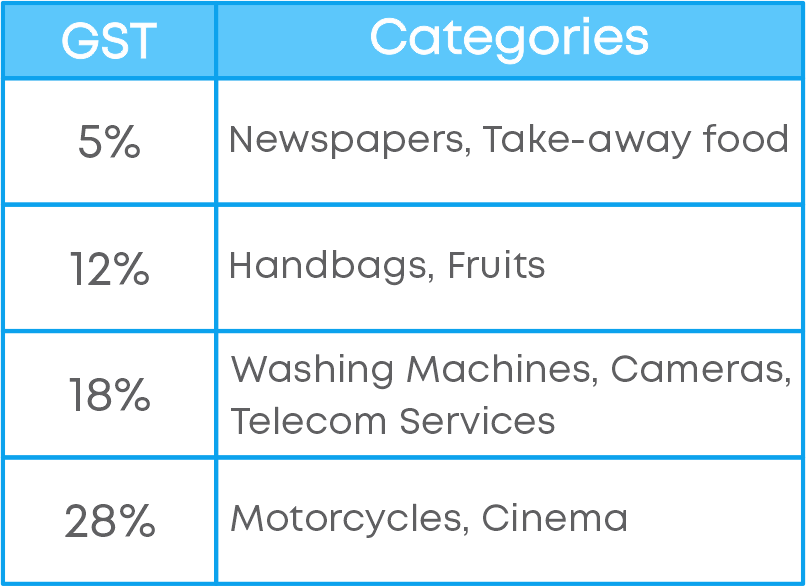If Anika buys a handbag of base price ₹1200, the amount she needs to pay, or the bill amount is base price + 8% of the base price.

= ₹1200 + 8% of ₹1200.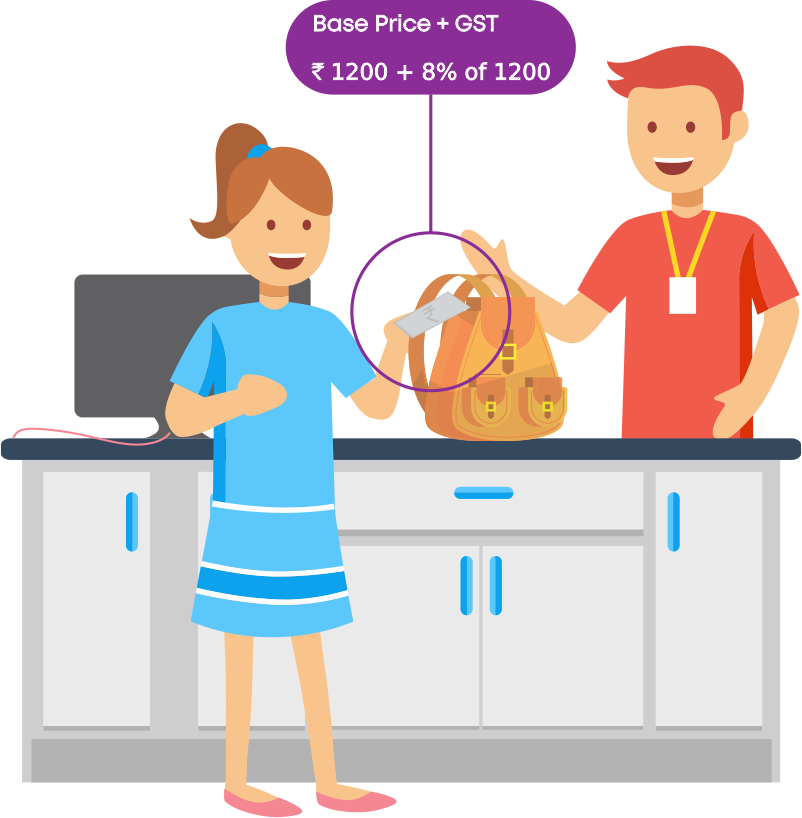The price of an item before GST (or tax) is added is called the base price. The price at which a customer buys a product including GST is called the bill amount.

Consider an example: Anshika went to a shop to buy a mixer. She asks the shopkeeper for the price of the mixer. The shopkeeper says its ₹5,000 without GST, and the GST charged on the mixer is 12%. How much money does Anshika need to pay to buy the mixer?

GST is always calculated on the base price.

To find the bill amount, follow these steps:

• Calculate the GST

GST = GST% of base price

• Add the GST to the base price

Bill amount = GST + base price

So, GST = 12% of ₹5,000 = ₹600

Bill amount = Base price + GST

= ₹5000 + ₹600

= ₹5600

Hence, Anshika needs to pay ₹5,600 to buy the mixer.

Consider another example: Sonali bought a cooler for ₹9,396 inclusive of GST at 8%. Find the base price of the cooler.

Let us consider the base price as ₹100. Then, the GST is 8% of ₹100, i.e., ₹8. Then, the bill amount will be ₹108.

When the bill amount is ₹108, then the base price is ₹100. Hence, when the bill amount is ₹9,396 what will be the base price?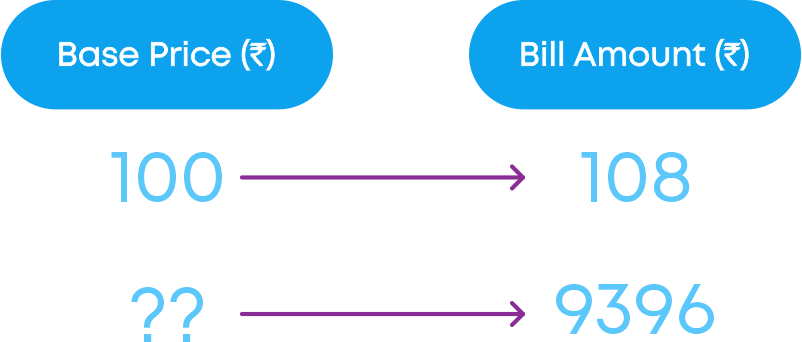By cross multiplying we get:

base price = ₹(100 × 100 x 9396 108 ) = ₹8700

Hence, the base price of the cooler is ₹8,700.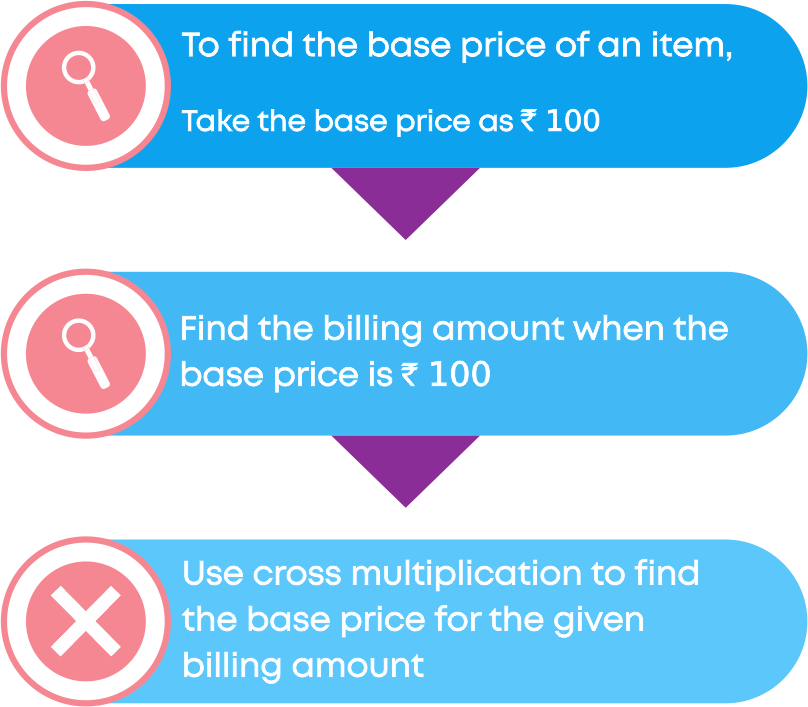Remember: GST is calculated as a percentage of the base price of an item.

Rate of GST / GST% = ( amount of GST base price x 100)%

Let us learn about something called ‘cess.’ Cess is the form of tax charged over and above the other tax. A cess is charged by the government to raise money for specific purposes. For e.g., the government charges educational cess to generate additional money for funding schools.

The government can charge cess for purposes such as disaster relief. For example, after the Kerala floods in 2018, the state government imposed a 1% calamity cess on GST.

#### Compound Interest

Rashmi had deposited ₹1,000 in her student bank account as fixed deposit for 3 years. The interest rate is 10% per annum.

After 3 years she gets an interest of ₹331, but when she calculates the interest, what she is supposed to get is ₹300. She gets confused and asks her father as to how she gets an interest of ₹331. Then her father explains to her that the banks never charge simple interest but they charge interest based on the compound interest. But Rashmi has no clue about compound interest. So let us help her understand what compound interest is.

Compound interest is the interest calculated on the principal and the interest accumulated over the previous period. It is different from the simple interest. The previous year's interest is not added to the principal while calculating the simple interest.

Let us understand the difference between compound and simple interest by taking an example.

Let us take the principal as ₹1,000 and rate of interest as 10% for 3 years.Under simple interest:

• We can see that for the second year and the third year the principal is ₹1000 only.
• Hence, the simple interest is calculated on principal only.

Under compound interest:

We can see that,

• For the second year: the principal is the amount at the end of the first year.
• For the third year: the principal is the amount at the end of the second year.

Hence, the compound interest is calculated on the principal and the interest previously earned.

If we need to find the amount paid for the loan taken for 15 years, do you think we should keep on calculating the interest for every year or is there any shorter way of calculating the CI?

There is a shorter way to calculate the amount. Let us understand the method.

• Let Principal = P
• Time = 'n' years
• Rate of interest = R

Let us calculate the amount and simple interest for the first year. The simple interest for the first year:

SI1 = P × R × T 100 = P × R × 1 100

The amount at the end of the first year:

A = P + SI1

A = P + P × R 100

A = P(1 + R 100 )

Let us calculate the amount and simple interest for the second year. We know that when the interest is compounded, the amount at the end of the year becomes the principal for the next year.

Principal for the second year = P(1 + R 100 ) = P2

The simple interest for the second year:

SI2 = P × R × T 100 = P × R × 1 100

Note: Here T = 1, as we are calculating the SI for one year.

• The amount at the end of the second year:

A = P2 + SI2

A = P2 + P2 × R × 1 100

A = P2(1 + R 100 ) (Since P2 = P(1 + R 100 ))

A = P(1 + R 100 ) × (1 + R 100 )

A = P(1 + R 100 )2

Proceeding in this way the amount at the end of 'n' years will be: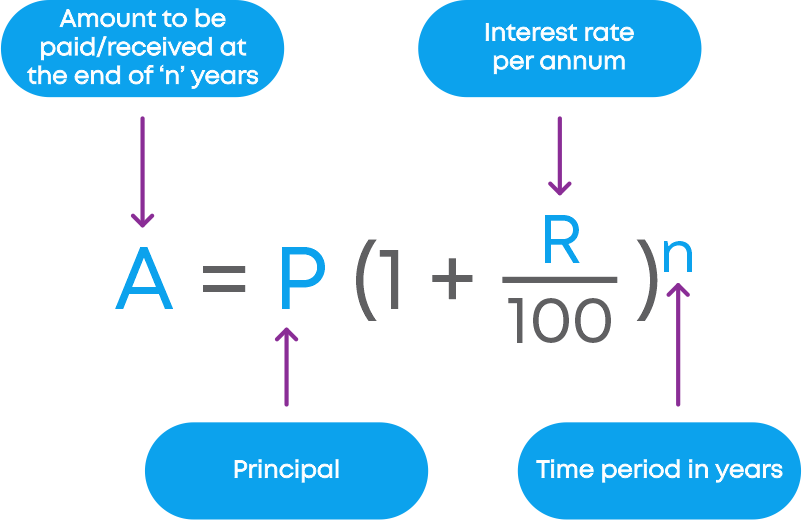The compound interest for ‘n’ years is: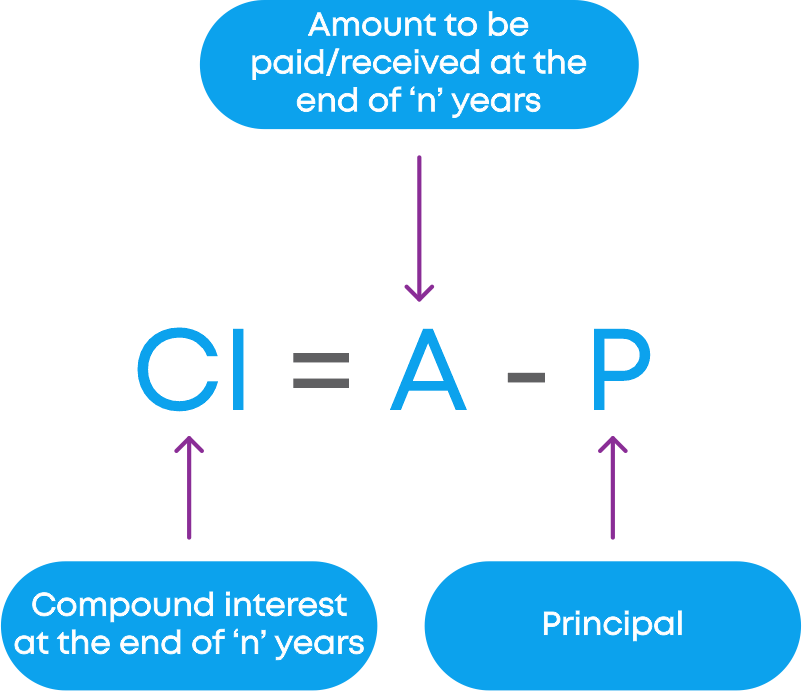A = P(1 + R 100 )n

Where n = number of years and R = rate per year

To find the CI when the interest is compounded annually,

CI = A - P

Let us understand how to find the compound interest.

Find the compound interest on ₹15,000 for 3 years at 10% per annum compounded annually.

Step 1: Identify the principal, rate of interest, and the time period.

Here we can see that,

• Principal P = ₹15,000
• Rate of interest = 10%
• Time period = 3 years

Step 2: Apply the formula.

To find the compound interest, first, we need to find the amount.

A = P(1 + R 100 )n

= 15000(1 + 10 100 )3

Then find the compound interest using the following formula CI = A – P

Step 3: Perform the calculation.Hence, the amount to be paid is ₹19,965. Now, let us find the compound interest,

CI = Amount – Interest

= ₹19965 – ₹15000

= ₹4965

Hence, the compound interest is ₹4,965.

To find the amount when the time period is given as a fraction, say n a b years, follow the given strategy.

• Divide the time period into 2 parts: The whole part (n) and the fractional part a b
• Calculate the amount at the end of ‘n’ years
• Calculate the simple interest for the remaining fractional part a b
• Add both amount and the simple interest to find the amount at the end of n a b years

Jyothi and Preethi have opened their bank accounts and deposited ₹1000 in it. They are discussing the interest rate that they have received at the end of the year.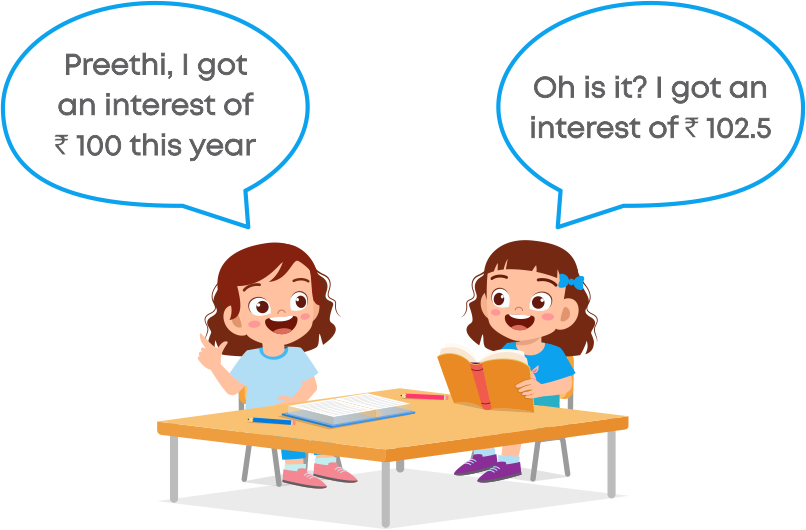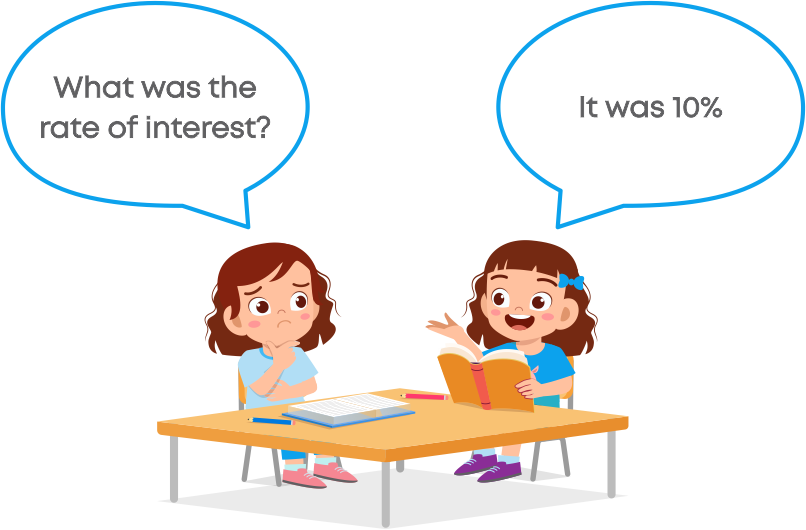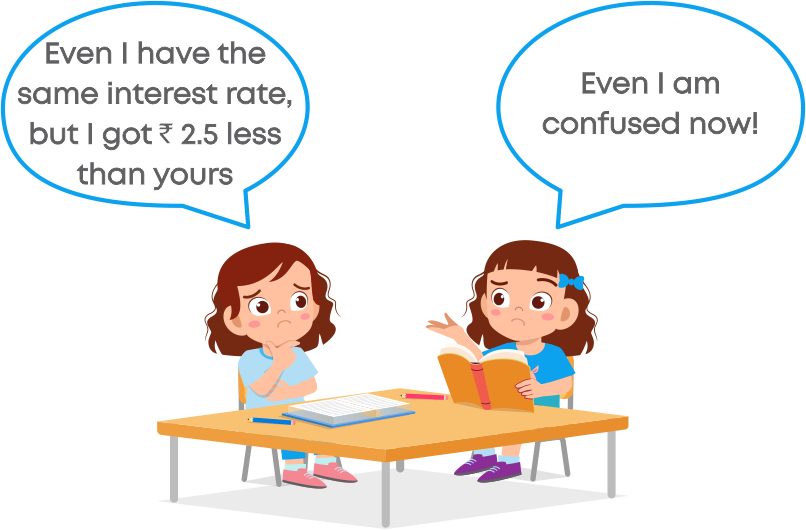Do you think is it possible to get different interest when the interest rate and principal are the same? Yes! In Jyothi’s case, the interest is compounded annually. That is, for every year the interest is calculated.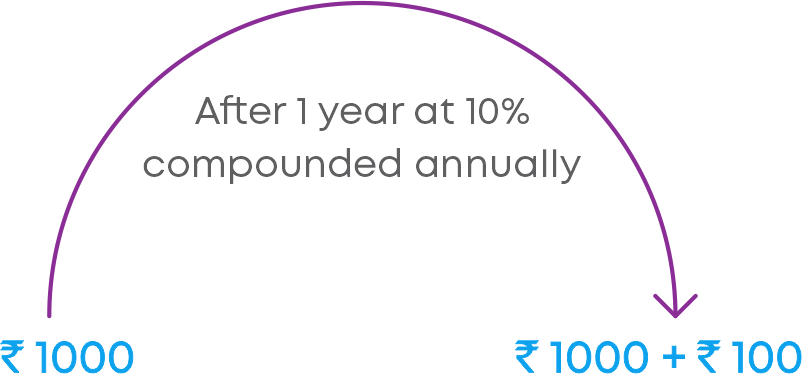In Preethi’s case, the interest is compounded half-yearly. That is, for every 6 months the interest is calculated.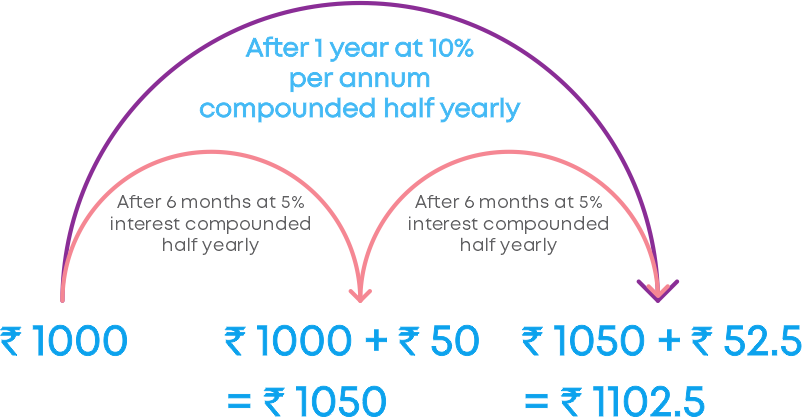That is why the interest is different even though the interest rate and principal are the same.

We know that when the interest is compounded annually:When the interest is calculated for every half year,

• R = Rate of interest per annum, hence, R 2 becomes the rate of interest for half year.• n = No. of years, but we need to count the number of half years, hence ‘n’ becomes 2n.• The amount at the end of 2n half years when the interest is compounded half-yearly,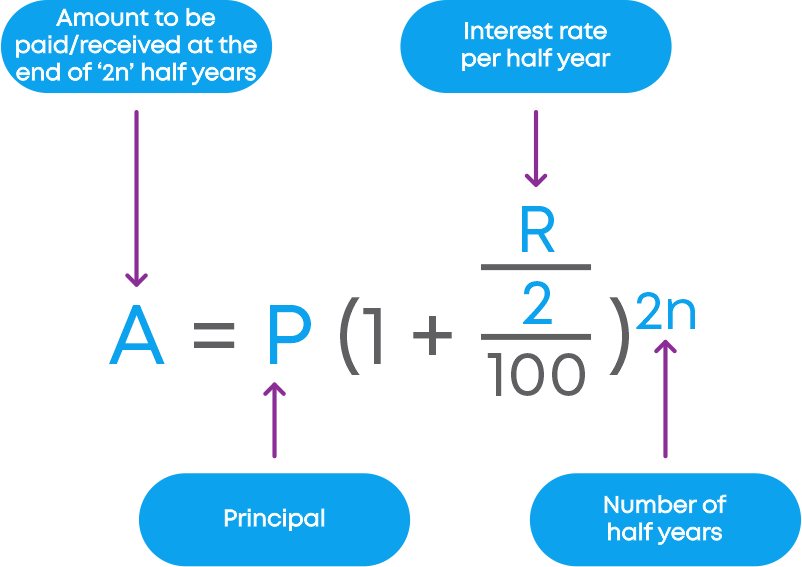• The compound interest at the end of 2n half years is,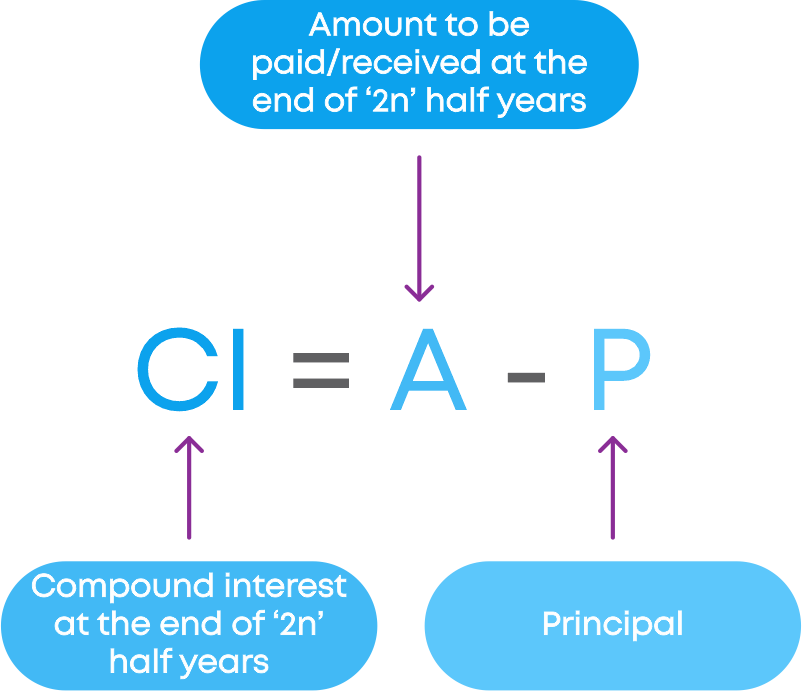So, to find the amount when the interest is compounded half-yearly,

A = P(1 + R 2 100 )2n

Where,

• 2n = number of half-years
• R 2 = Half-year rate

To find the CI when the interest is compounded half-yearly, CI = A – P.

When the interest is compounded quarterly,

• R = Rate of interest per annum, hence, R 4 becomes the rate of interest for a quarter year• n = No. of years, but we need to count the number of quarter years, hence ‘n’ becomes 4nTime period = 4n = no. of quarters

• The amount when the interest is compounded quarterly,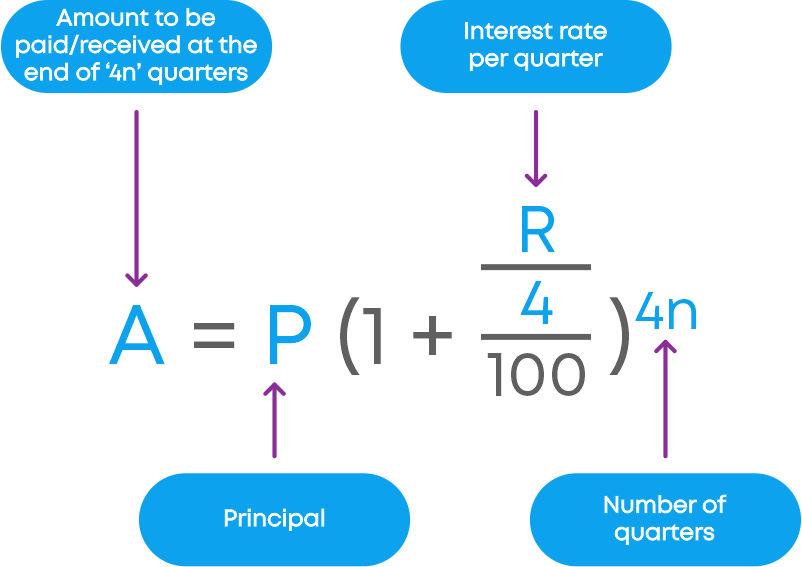• The CI when the interest is compounded quarterly,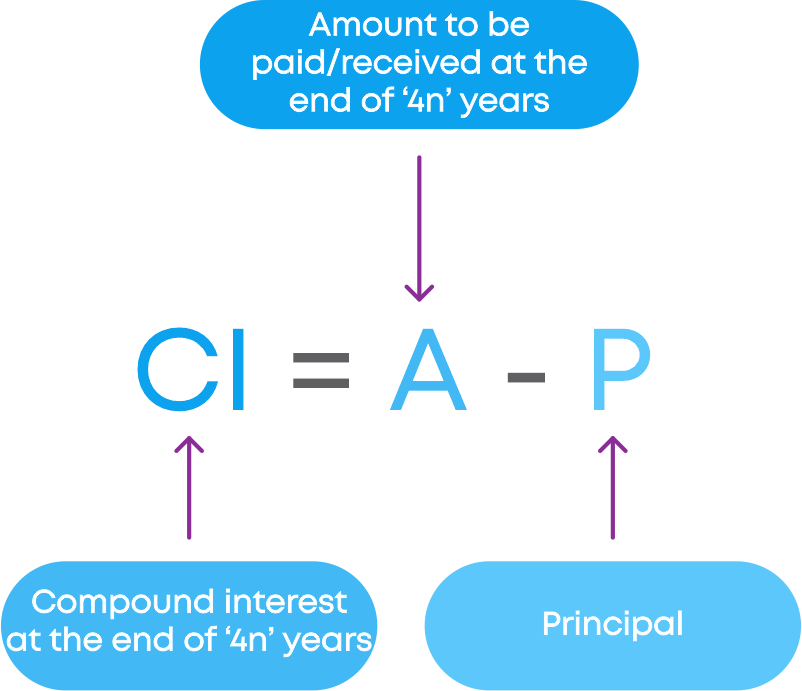So, the amount to be paid/received when the interest is compounded quarterly:

A = P(1 + R 4 100 )4n

Where,

• R 4
• 4n = no. of quarters

Compound interest when the interest is compounded quarterly, CI = A – P.

#### Compound Interest

There are some situations where we could use the formula for calculation of the amount in CI.

• Increase or decrease in population
• Growth of the bacteria count if the rate of growth is known
• Value of the item if the price increases or decreases

Example: A survey was conducted in a village. From the survey, it was shown that every year the population of the village increases by 5%. If the present population of the village is 10,000, how do we find the population of the village after 2 years?

• Population at the end of the first year P1 = 10000 + 5% of 10000
• The population at the end of the second year = Population at the end of the first year + 5% of P1

But if we are asked to find the population at the end of 10 years, then we need to find an efficient way to do it. Do you think can we use the compound interest formula to find the population at the end of 10 years? Yes! We can see that the population is increasing in compounded form. Hence, we can use the formula of CI to find an increase in the population.

If the population is increasing in the compounded form:

• The population at the end of ‘n’ years = P(1 + R 100 )n

Where P = Present population,

R = Rate of increase

n= Number of years

If the population is decreasing in the compounded form:

• The population at the end of ‘n’ years = P(1 - R 100 )n

Where P = Present population,

R = Rate of decrease

n= Number of years

Hence, we can calculate the population after 10 years = 10000(1 + 5 100 )10

Anshika bought a TV for ₹28,000 two years ago. Now she wants to sell that TV and purchase a new one. Do you think will she get ₹28,000 if she sells her TV? No! Anshika used that TV for 2 years, so it is not a new TV anymore. Hence, the value of that TV will be less than ₹28,000.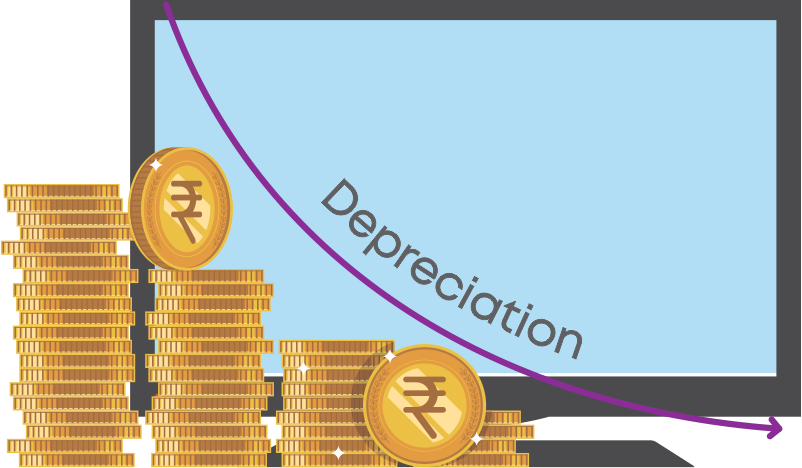The decrease in the value of items over a period of time is known as depreciation. Depreciation happens due to usage and ageing of an item.

Remember the formula: if V0 is the value of the item at present, and R is the rate of depreciation, then the value of the item after ‘n’ years

V = V0(1 - R 100 )n

Example: A bicycle was bought at ₹8,000. Its value depreciates at the rate of 10% per annum. Find the value of the bicycle after 2 years.

The cost of the bicycle was ₹8,000. The value of the bicycle decreases at the rate of 10% per annum. We need to find the value of the bicycle after 2 years.

How do we find the value of the bicycle after 2 years? Can we use the formula of compound interest? Yes! There is a 10% decrease in the value of the bicycle every year. So, it is decreasing in compounded form.

Hence, we use the CI formula to calculate the value of the bicycle after 2 years

• Let us take V as the value of the bicycle after 2 years.
• V0 as the present value of the bicycle.
• R is the rate of depreciation.
• ‘n’ is the number of years

Using the formula for compound interest we write the value of the bicycle after 2 years:

V = ₹8000(1 - 10 100 )2

= ₹8000( 9 10 )2

= ₹6480

Hence, the value of the bicycle after 2 years is ₹6,480.

#### Common Errors

The following are topics in which students make common mistakes when dealing with comparing quantities:

• 1. Profit or loss per cent, Discount per cent and GST per cent
• 2. How to calculate compound interest?
• 3. How to calculate the compound interest when interest is compounded half-yearly?
• 4. How to calculate compound interest when interest is compounded quarterly?

#### Profit Or Loss Per Cent, Discount Per Cent And GST Per Cent

• The profit or loss per cent is always calculated on the cost price of an item, not the selling price.
• The discount per cent is always calculated on the marked price of an item, not the selling price.
• The GST per cent is always calculated on the base price of an item, not the cost price.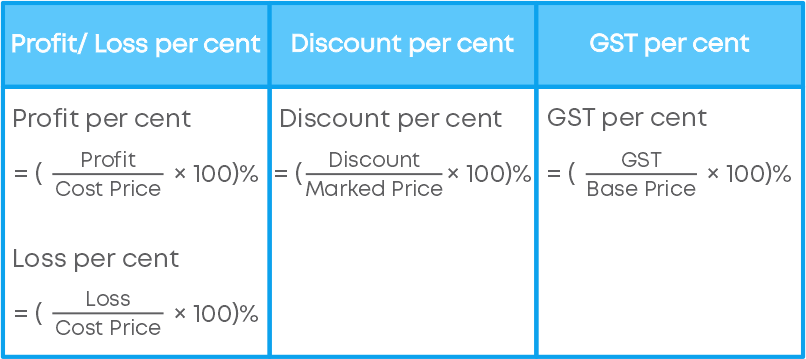#### How To Calculate Compound Interest?

To find the compound interest, we first find the amount by using the formula,

A = P × (1 + R 100 )n

This formula gives the amount to be paid or received at the end of ‘n’ years. Now to find the compound interest:

Compound interest = Amount – Principle.

#### How To Calculate The Compound Interest When Interest Is Compounded Half-Yearly?

To find the compound interest, when the interest is compounded half-yearly, we first use the formula:

A = P × (1 + R 2 100 )2n

For e.g., Find CI, Principal P = ₹2000, Rate of interest R = 8% and Time period n = 2 years. Since the interest is compounded half-yearly,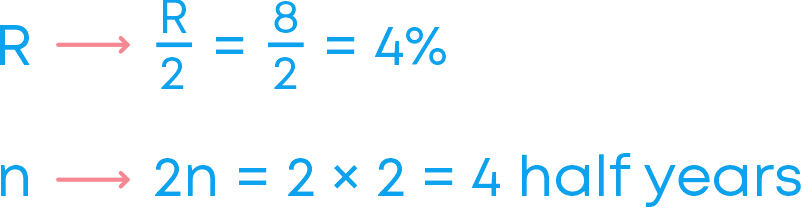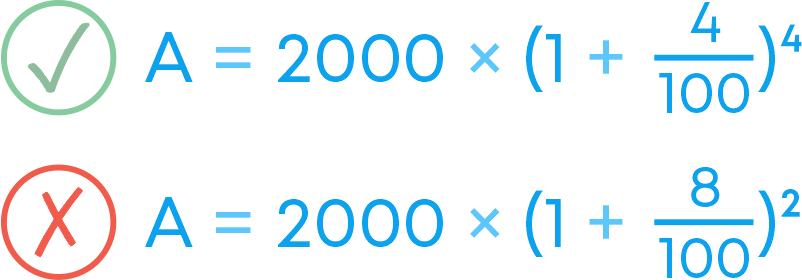This formula gives the amount to be paid or received at the end of ‘2n’ half-years. Now to find the compound interest:

Compound interest = Amount – Principle.

#### How To Calculate Compound Interest When Interest Is Compounded Quarterly?

To find the compound interest, when the interest is compounded quarterly, we first use the formula:

A = P × (1 + R 4 100 )4n

For e.g., Find CI, Principal P = ₹2000, Rate of interest R = 8% and Time period n = 2 years. Since the interest is compounded quarterly,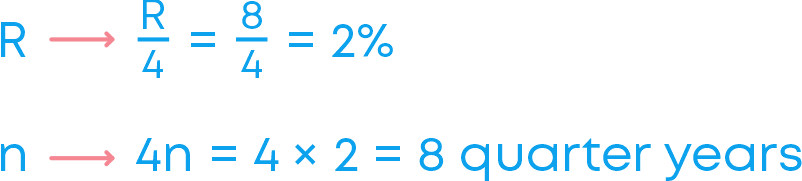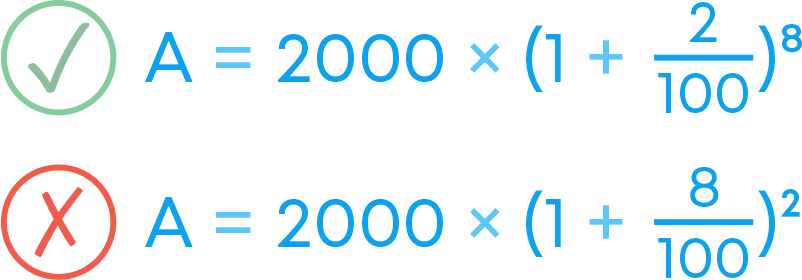This formula gives the amount to be paid or received at the end of ‘4n’quarter- years. Now to find the compound interest:

Compound interest = Amount – Principle.

#### Conclusion

Now that you have learnt about comparing quantities, ratios, and percentages, can you solve this riddle?

The ratio of sugar to flour for cooking a cake is 1 2 to 2. What percent of the cake is flour?

(Assume that there is only sugar and flour in the cake)#### Jyothi

Author

A math enthusiast and an educationist, Jyothi has a strong academic background and has demonstrated an impressive history of working in the education industry. Jyothi is skilled in developing and reviewing lesson plans, learning content, assessments, and video story boards and scripts.